# Present Value Formulas, Tables and Calculators

The easiest and most accurate way to calculate the present value of any future amounts (single amount, varying amounts, annuities) is to use an electronic financial calculator or computer software. Some electronic financial calculators are now available for less than \$35.

IMPORTANT! Understand that each financial calculator operates differently and may operate in a manner that is different from our Explanation. Be certain to read and thoroughly understand the directions before operating any calculator or computer software. One incorrect assumption, one incorrect input, or the use of a button that works differently from what you assumed, will mean an incorrect answer—and that could have a significant financial consequence!

If you don't have access to an electronic financial calculator or software, an easy way to calculate present value amounts is to use present value tables (PV tables). PV tables cannot provide the same level of accuracy as financial calculators or computer software because the factors used in the tables are rounded off to fewer decimal places. In addition, they usually contain a limited number of choices for interest rates and time periods. Despite this, present value tables remain popular in academic settings because they are easy to incorporate into a textbook. Because of their widespread use, we will use present value tables for solving our examples.

Behind every table, calculator, and piece of software, are the mathematical formulas needed to compute present value amounts, interest rates, the number of periods, and the future value amounts. We will, at the outset, show you several examples of how to use the present value formula in addition to using the PV tables.

Except for minor differences due to rounding, answers to equations below will be the same whether they are computed using a financial calculator, computer software, PV tables, or the formulas.

## Calculating the Present Value of a Single Amount (PV)

In this section we will demonstrate how to find the present value of a single future cash amount, such as a receipt or a payment. We'll refer to the present value of a single amount as PV.

### Exercise #1

Let's assume we are to receive \$100 at the end of two years. How do we calculate the present value of the amount, assuming the interest rate is 8% per year compounded annually?

The following timeline depicts the information we know, along with the unknown component (PV):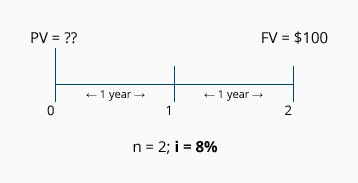Calculation Using the PV Formula

The present value formula for a single amount is: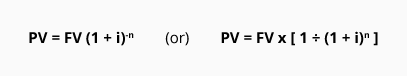Using the second version of the formula, the solution is: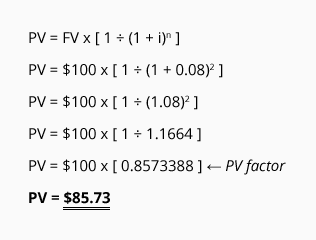The answer, \$85.73, tells us that receiving \$100 in two years is the same as receiving \$85.73 today, if the time value of money is 8% per year compounded annually. ("Today" is the same concept as "time period 0.")

Calculation Using a PV of 1 Table

It's common for accounting and finance textbooks to provide present value tables to use in calculating present value amounts. In a PV of 1 table, each column heading displays an interest rate (i), and the row indicates the number of periods into the future before an amount will occur (n). At the intersection of each column and row is the correlating present value of 1 (PV of 1) factor. The PV of 1 factor tells us what the present value will be, at time period 0, for a single amount of \$1 at the end of time period (n). Click the following to see a present value of 1 table: PV of 1 Table.

The PV of 1 table has two limitations: (1) values are rounded (ours has the rounding to three decimal places for ease of use) and thus the table sacrifices a bit of accuracy, and (2) the table displays only a limited number of choices for rates and years.

Once you determine the PV of 1 factor from the table, simply use it to substitute for the following term in the PV formula: [ 1 ÷ (1 + i)n ]Using the data presented in Exercise #1, we can solve for the present value of receiving \$100 at the end of two years, when discounted by an interest rate of 8% compounded annually: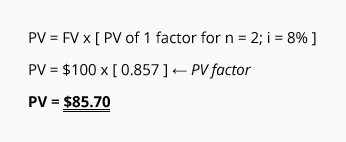Because the PV of 1 table had the factors rounded to three decimal places, the answer (\$85.70) differs slightly from the amount calculated using the PV formula (\$85.73). In either case, what the answer tells us is that \$100 at the end of two years is the equivalent of receiving approximately \$85.70 today (at time period 0) if the time value of money is 8% per year compounded annually.

### Exercise #2

We need to calculate the present value (the value at time period 0) of receiving a single amount of \$1,000 in 20 years. The interest rate for discounting the future amount is estimated at 10% per year compounded annually.

The following timeline depicts the information we know, along with the unknown component (PV):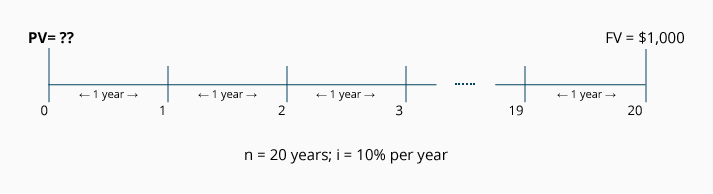Calculation Using the PV of 1 Formula
Using the formula to determine the present value, we have: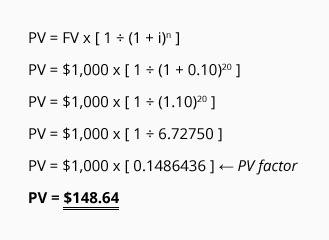The answer tells us that receiving \$1,000 in 20 years is the equivalent of receiving \$148.64 today, if the time value of money is 10% per year compounded annually.

Calculation Using a PV of 1 Table
Use the PV of 1 table to find the (rounded) present value factor at the intersection of n = 20 and i = 10%. To calculate the present value of receiving \$1,000 at the end of 20 years with a 10% interest rate, insert the factor into the formula: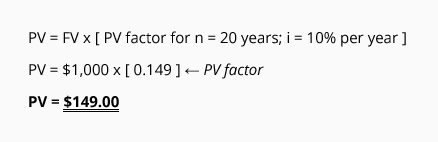We see that the present value of receiving \$1,000 in 20 years is the equivalent of receiving approximately \$149.00 today, if the time value of money is 10% per year compounded annually.

### Exercise #3

What is the present value of receiving a single amount of \$5,000 at the end of three years, if the time value of money is 8% per year, compounded quarterly?

The following timeline depicts the information we know, along with the unknown component (PV):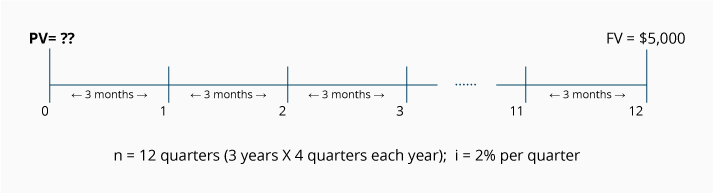Notice that the timeline shows n = 12, because there are 12 quarters in the three-year period. Because the time periods are three months long, the rate for discounting is i = 2% (the quarterly rate that results from the annual rate of 8% divided by the four quarters in each year).

Calculation Using the PV Formula
Using the formula to determine the present value, we have: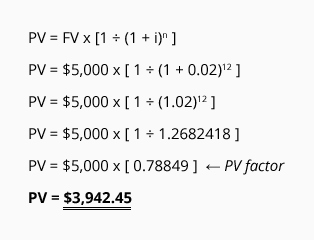The answer tells us that receiving \$5,000 three years from today is the equivalent of receiving \$3,942.45 today, if the time value of money has an annual rate of 8% that is compounded quarterly.

Calculation Using a PV of 1 Table
The present value of receiving \$5,000 at the end of three years when the interest rate is compounded quarterly, requires that (n) and (i) be stated in quarters. Use the PV of 1 Table to find the (rounded) present value figure at the intersection of n = 12 (3 years x 4 quarters) and i = 2% (8% per year ÷ 4 quarters). Insert the factor into the formula: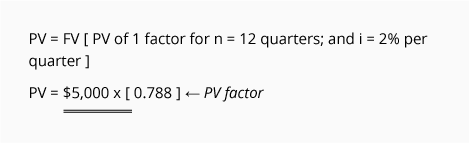We see that the present value of receiving \$5,000 three years from today is approximately \$3,940.00 if the time value of money is 8% per year, compounded quarterly.

### Exercise #4

What is the present value of receiving a single amount of \$10,000 at the end of five years, if the time value of money is 6% per year, compounded semiannually?

The following timeline depicts the information we know, along with the unknown component (PV):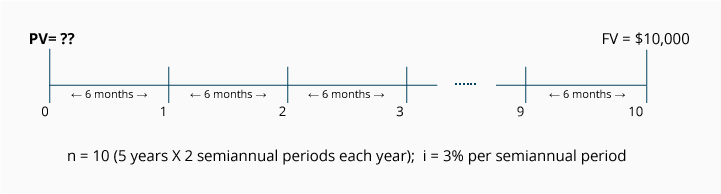Notice that the timeline shows n = 10, because there are 10 six-month (or semiannual) periods in five-years time. Because the compounding occurs semiannually, the rate for discounting is i = 3% per six-month period (the annual rate of 6% divided by the two semiannual periods in each year).

Calculation Using the PV Formula
Using the formula to determine the present value, we have: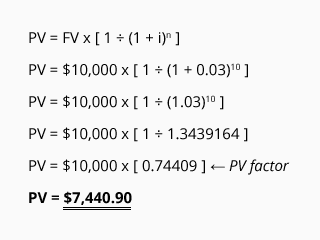The answer tells us that receiving \$10,000 five years from today is the equivalent of receiving \$7,440.90 today, if the time value of money has an annual rate of 6% compounded semiannually.

Calculation Using a PV of 1 Table
The present value of receiving \$10,000 at the end of five years when the compounding is semiannual, requires that n = 10 (5 years X 2 semiannual periods per year) and that i = 3% (6% per year ÷ two semiannual periods in each year). Use the PV of 1 Table to find the (rounded) present value factor at the intersection of n = 10 and i = 3%. Insert the factor into the formula: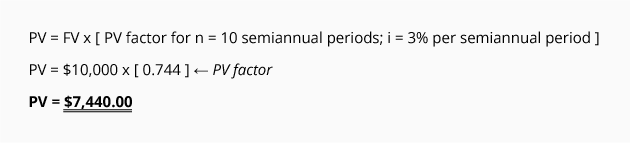We see that the present value of receiving \$10,000 five years from today is the equivalent of receiving approximately \$7,440.00 today, if the time value of money has an annual rate of 6% compounded semiannually.